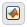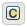## Supported Operations for Vectors and Matrices

Stateflow® charts in Simulink® models have an action language property that defines the syntax that you use to compute with vectors and matrices. The action language properties are:

•MATLAB® as the action language.

•C as the action language.

For more information, see Differences Between MATLAB and C as Action Language Syntax.

### Indexing Notation

In charts that use MATLAB as the action language, refer to elements of a vector or matrix by using one-based indexing delimited by parentheses. Separate indices for different dimensions with commas.

In charts that use C as the action language, refer to elements of a vector or matrix by using zero-based indexing delimited by brackets. Enclose indices for different dimensions in their own pair of brackets.

Example

MATLAB as the Action Language

C as the Action Language
The first element of a vector `V``V(1)``V`
The `i`th element of a vector `V``V(i)``V[i-1]`
The element in row `4` and column `5` of a matrix `M``M(4,5)``M`
The element in row `i` and column `j` of a matrix `M``M(i,j)``M[i-1][j-1]`

### Binary Operations

This table summarizes the interpretation of all binary operations on vector and matrix operands according to their order of precedence (1 = highest, 3 = lowest). Binary operations are left associative so that, in any expression, operators with the same precedence are evaluated from left to right. Except for the matrix multiplication and division operators in charts that use MATLAB as the action language, all binary operators perform element-wise operations.

Operation

Precedence

MATLAB as the Action Language

C as the Action Language

`a * b`

1

Matrix multiplication.

Element-wise multiplication. For matrix multiplication, use the `*` operation in a MATLAB function. See Perform Matrix Arithmetic by Using MATLAB Functions.

`a .* b`

1

Element-wise multiplication.

Not supported. Use the operation ```a * b```.

`a / b`

1

Matrix right division.

Element-wise right division. For matrix right division, use the `/` operation in a MATLAB function. See Perform Matrix Arithmetic by Using MATLAB Functions.

`a ./ b`

1

Element-wise right division.

Not supported. Use the operation ```a / b```.

`a \ b`

1

Matrix left division.

Not supported. Use the `\` operation in a MATLAB function. See Perform Matrix Arithmetic by Using MATLAB Functions.

`a .\ b`

1

Element-wise left division.

Not supported. Use the `.\` operation in a MATLAB function. See Perform Matrix Arithmetic by Using MATLAB Functions.

`a + b`

2

`a - b`

2

Subtraction.

Subtraction.

`a == b`

3

Comparison, equal to.

Comparison, equal to.

`a ~= b`

3

Comparison, not equal to.

Comparison, not equal to.

`a != b`

3

Not supported. Use the operation ```a ~= b```.

Comparison, not equal to.

`a <> b`

3

Not supported. Use the operation ```a ~= b```.

Comparison, not equal to.

### Unary Operations and Actions

This table summarizes the interpretation of all unary operations and actions on vector and matrix operands. Unary operations:

• Have higher precedence than the binary operators.

• Are right associative so that, in any expression, they are evaluated from right to left.

• Perform element-wise operations.

Example

MATLAB as the Action Language

C as the Action Language

`~a`

Logical NOT. For bitwise NOT, use the `bitcmp` function.

• Bitwise NOT (default). Enable this operation by selecting the Enable C-bit operations chart property.

• Logical NOT. Enable this operation by clearing the Enable C-bit operations chart property.

`!a`

Not supported. Use the operation `~a`.

Logical NOT.

`-a`

Negative.

Negative.

`a++`

Not supported.

Increment all elements of the vector or matrix. Equivalent to `a = a+1`.

`a--`

Not supported.

Decrement all elements of the vector or matrix. Equivalent to `a = a-1`.

### Assignment Operations

This table summarizes the interpretation of assignment operations on vector and matrix operands.

Operation

MATLAB as the Action Language

C as the Action Language

`a = b`

Simple assignment.

Simple assignment.

`a += b`

Not supported. Use the expression ```a = a+b```.

Equivalent to ```a = a+b```.

`a -= b`

Not supported. Use the expression ```a = a-b```.

Equivalent to ```a = a-b```.

`a *= b`

Not supported. Use the expression ```a = a*b```.

Equivalent to ```a = a*b```.

`a /= b`

Not supported. Use the expression ```a = a/b```.

Equivalent to ```a = a/b```.

#### Assign Values to Individual Elements of a Matrix

You can assign a value to an individual entry of a vector or matrix by using the indexing syntax appropriate to the action language of the chart.

Example

MATLAB as the Action Language

C as the Action Language
Assign the value `10` to the first element of the vector `V`.`V(1) = 10;``V = 10;`
Assign the value 77 to the element in row 2 and column 9 of the matrix `M`.`M(2,9) = 77;``M = 77;`

#### Assign Values to All Elements of a Matrix

In charts that use MATLAB as the action language, you can use a single action to specify all of the elements of a vector or matrix. For example, this action assigns each element of the 2-by-3 matrix `A` to a different value:

`A = [1 2 3; 4 5 6];`

In charts that use C as the action language, you can use scalar expansion to set all of the elements of a vector or matrix to the same value. Scalar expansion converts scalar data to match the dimensions of vector or matrix data. For example, this action sets all of the elements of the matrix `A` to `10`:

`A = 10;`

Scalar expansion applies to all graphical, truth table, MATLAB, and Simulink functions. Suppose that you define the formal arguments of a function `f` as scalars. This table describes the rules of scalar expansion for the function call ```y = f(u)```.

Output `y`Input `u`Result
ScalarScalarNo scalar expansion occurs.
ScalarVector or matrixThe chart generates a size mismatch error.
Vector or matrixScalar

The chart uses scalar expansion to assign the scalar output value of `f(u)` to every element of `y`:

`y[i][j] = f(u)`

Vector or matrixVector or matrix

The chart uses scalar expansion to compute an output value for each element of `u` and assign it to the corresponding element of `y`:

`y[i][j] = f(u[i][j])`
If `y` and `u` do not have the same size, the chart generates a size mismatch error.

For functions with multiple outputs, the same rules apply unless the outputs and inputs are all vectors or matrices. In this case, the chart generates a size mismatch error and scalar expansion does not occur.

Charts that use MATLAB as the action language do not support scalar expansion.

### Perform Matrix Arithmetic by Using MATLAB Functions

In charts that use C as the action language, the operations `*` and `/` perform element-wise multiplication and division. To perform standard matrix multiplication and division in a C chart, use a MATLAB function.

Suppose that you want to perform these operations on the square matrices `u1` and `u2`:

• Compute the standard matrix product ```y1 = u1 * u2```.

• Solve the equation `u1 * y2 = u2`.

• Solve the equation `y3 * u1 = u2`.

To complete these calculations in a C chart, add a MATLAB function that runs this code:

```function [y1, y2, y3] = my_matrix_ops(u1, u2) %#codegen y1 = u1 * u2; % matrix multiplication y2 = u1 \ u2; % matrix division from the right y3 = u1 / u2; % matrix division from the left```
Before calling the function, specify the properties for the input and output data, as described in Set Data Properties.

In charts that use MATLAB as the action language, the operations `*`, `/`, and `\` perform standard matrix multiplication and division. You can use these operations directly in state and transition actions.